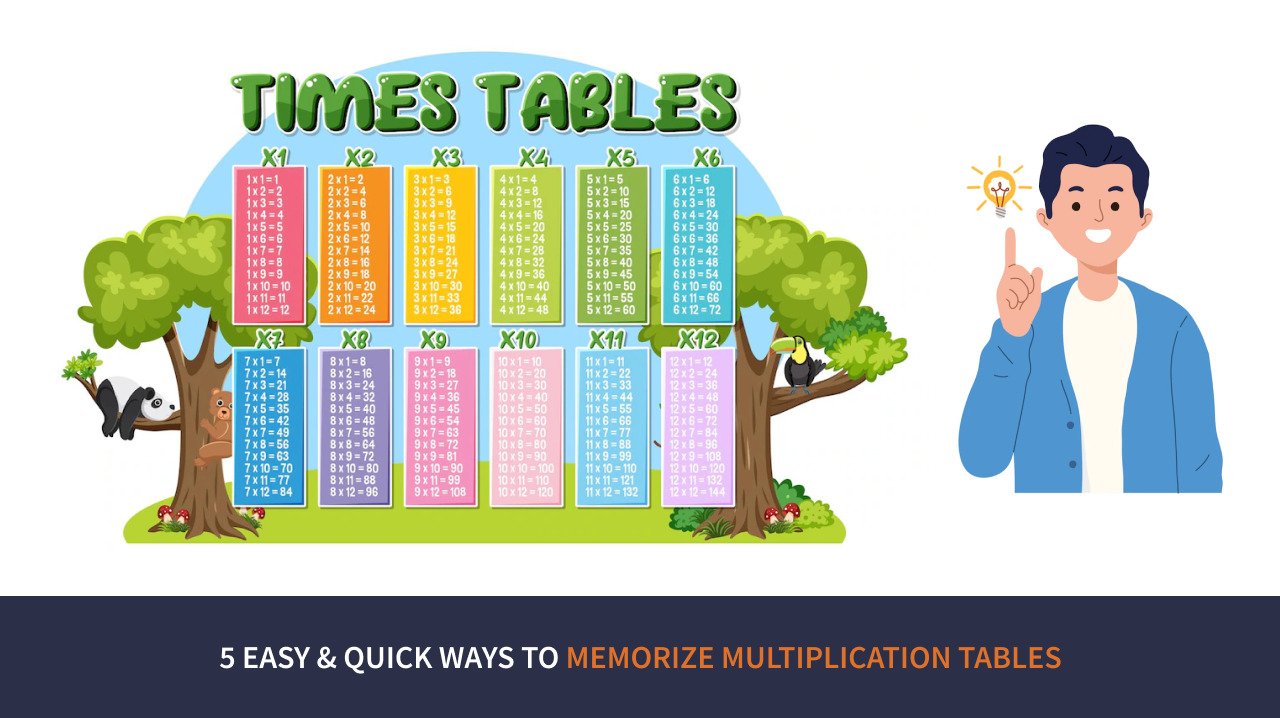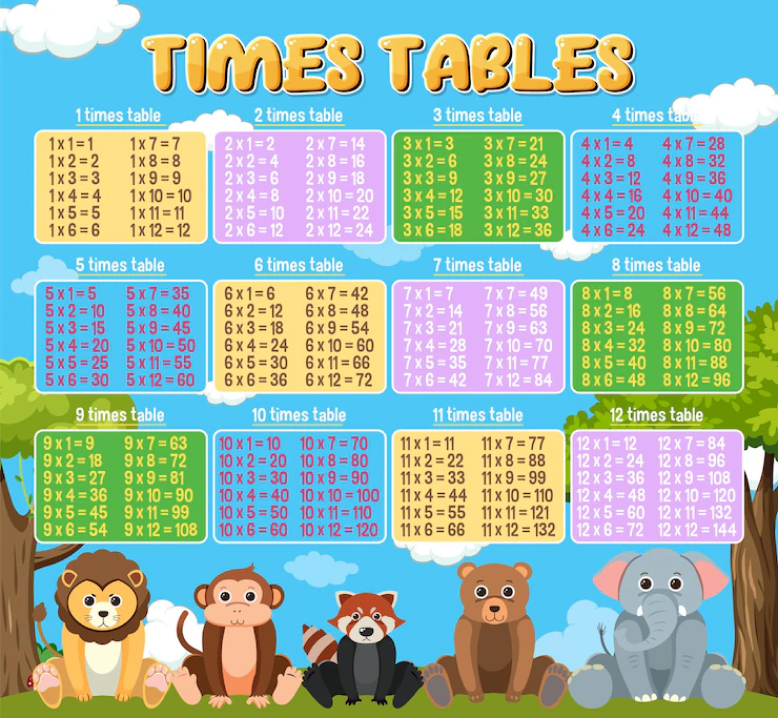# 5 Easy & Quick Ways to Memorise Multiplication TablesMultiplication is one of the most basic operations required to perform calculations of basic mathematical problems and is used in performing advanced mathematical problems. Multiplication Tables play an essential role in the primary phase of every child’s education, and rightly so. We are always asked to memorise multiplication tables but are never introduced to the various methods developed over time to remember these tables.

This article explains the various practical methods experimented with and developed to memorise multiplication tables. Before we understand the different techniques to learn tables, let us understand why memorising tables is essential.

As mentioned above, multiplication tables are basic operations, and children must recall answers to multiplication problems. Recalling the answers quickly is essential to perform advanced mathematical problems.

With the importance of multiplication tables discussed, let us now understand a few difficulties associated with memorising multiplication tables.

A few common problems students face are recalling answers to specific tables, the speed at which the answers are remembered and understanding the various methods used to derive solutions.

## Uses of Multiplication Table

Learning multiplication tables can be used in solving many day-to-day problems. Some of the few areas where the concept of multiplication can be applied are-

• While calculating prices of various groceries and other commodities.
• While mass, area and volume of multiple objects.

These are just a few basic day-to-day applications of multiplication, and there are many more applications that the students can explore.

### How To Learn Tables?

It is amazing how many methods are available to address the question, “ How to learn tables?”.  Below, we look at various multiplication facts that have helped develop techniques to remember multiplication tables.

## Method 1: Visualise Pattern

Visualise Pattern is a basic method that can be used to learn the multiplication of tables effectively yet easily. In this method, students are advised to imagine the problem with objects of daily use to understand the concept better. This method promotes a fun way to learn tables that can be included by the teachers and student effectively in a student’s study timetable

## Method 2: Mnemonics and Memory Tricks

Memorising tables can be boring and challenging to children, which is why we have noted a few tricks that will help students remember the tables easily. Here are a few tricks that might help your child remember the tables in a fun way –

• For 2X – Teach your children to understand this table; we must add the number twice. For example, 2×3 = 6; 3+3=6
• For 4X, it is important to teach your children that it is as good as doubling the answer derived by adding the given number twice and adding the number by itself. For example, 4X5=20; 5+5=10, 10+10= 20.
• For 5X, a foolproof method to know if the answer is right is to check if the answer contains 5 or 0 in its units place.
• For 8X, it is as simple as doubling three times. For example, 8X3= 24; 3+3=6, 6+6 =12, 12+12 = 24.
• For 10X, just tell your child to add the 0 to the number 10 is multiplied by. For example, – 10X2= 20, 10X9=90.

This method helps in time management and allows students to arrive at answers effectively and swiftly.

## Method 3: Chunking and Grouping

This is a more advanced method of understanding the concept of multiplication and can be learned in tandem with understanding the concept of division. It is also a method that showcases the relationship between multiplication and division. For example, 2×4=8 and 8/4, where 4 is the divisor, and 8 is the dividend = 2.

## Method 4: Times Tables Songs and Rhythms

Songs and rhythms are a great way to learn anything, and this method can be used to learn multiplication tables in a fun way! Songs and rhythms call for the use of memory power and also significantly answer the ever-elusive question, “ How to memorise multiplication tables?”. Here is a list of a few songs and rhythms to learn multiplication tables-

• The 2 Times Table Song.
• You’ve got a friend in Three.
• The ants go marching.

You can also come up with your song and start learning multiplication tables in a fun way!

## Method 5: Regular Practice and Games

Apart from the above-mentioned tips and tricks, regular practice and playing games based on real-life application of multiplication is also a very effective way of learning multiplication tables.